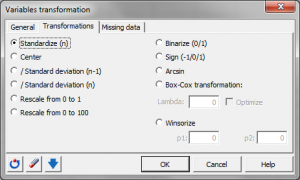# Variable transformations

Variable transformations have many purposes. For example, they may help you normalize your data. Do it in Excel using the XLSTAT statistical software.## Use of variable transformation

Variable transformation is often necessary to get a more representative variable for the purpose of the analysis. It can also be used simply to let your variable's distribution get closer to a normal distribution (notice that this does not work systematically).

Deciding on the appropriate transformation will often improve the quality of your results.

## XLSTAT variable transformation functions

This function allows you to transform a quantitative variable using many different analytical functions.

XLSTAT provides the following analytical functions:

• Standardize (n-1) To standardize the variables using the unbiased standard deviation.
• Standardize (n) To standardize the variables using the biased standard deviation.
• Center To center the variables.
• 1/ Standard deviation (n-1) To divide the variables by their unbiased standard deviation.
• 1/ Standard deviation (n) To divide the variables by their biased standard deviation.
• Rescale from 0 to 100
• Binarize (0/1) To convert all values that are not 0 to 1, and leave the 0s unchanged.
• Sign (-1/0/1) To convert all values that are negative to -1, all positive values to 1, and leave the 0s unchanged.
• Arcsin
• Box-Cox transformation To improve the normality of the sample.
• Winsorize To remove data that are not within an interval defined by two percentiles.
• Johnson transformation To improve the normality of the sample.### analyze your data with xlstat

14-day free trial

Included in

Related features